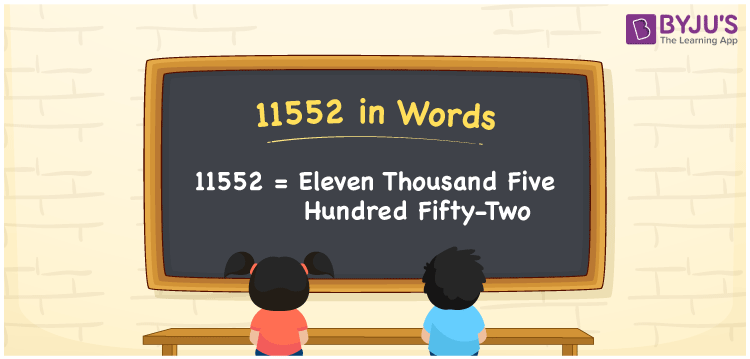# 11552 in Words

We can write 11552 in words as Eleven thousand five hundred fifty-two. If you bought a new Vacuum cleaner that cost Rs. 11552, then you can say, “I bought a new Vacuum cleaner worth Eleven thousand five hundred fifty-two rupees”. We can easily convert the number 11552 into words using a place value chart.

 11552 in words Eleven thousand five hundred fifty-two Eleven thousand five hundred fifty-two in Numbers 11552

## 11552 in English Words

Generally, we write numbers in words using the English alphabet. Thus, we spell 11552 in English words as “Eleven thousand five hundred fifty-two”.## How to Write 11552 in Words?

As we can see, 11552 is a five-digit number so, let’s create a place value chart with five columns, as shown below.

 Ten thousands Thousands Hundreds Tens Ones 1 1 5 5 2

Here, ones = 2, tens = 5, hundreds = 5, thousands = 1, ten thousands = 1

The above numbers can be expanded as follows.

1 × Ten thousand + 1 × Thousand + 5 × Hundred + 5 × Ten + 2 × One

= 1 × 10000 + 1 × 1000 + 5 × 100 + 5 × 10 + 2 × 1

= 10000 + 1000 + 500 + 50 + 2

= Ten Thousand + One thousand + Five hundred + Fifty + Two

= Eleven thousand + Five hundred Fifty-two

= Eleven thousand five hundred fifty-two

11552 is a natural number that is the successor of 11551 and predecessor of 11553.

11552 in words – Eleven thousand five hundred fifty-two

Is 11552 an odd number? – No

Is 11552 an even number? – Yes

Is 11552 a prime number? – No

Is 11552 a composite number? – Yes

Is 11552 a perfect square number? – No

Is 11552 a perfect cube number? – No

## Frequently Asked Questions on 11552 in Words

Q1

### How do you write 11552 in words?

We can write the number 11552 in words as Eleven thousand five hundred fifty-two.
Q2

### How to write Rs. 11552 in words on a cheque?

On a cheque, we generally write Rs. 11552 in words as “Eleven thousand five hundred fifty-two rupees only”.
Q3

### What is the number name for 11552?

The number name for 11552 is Eleven thousand five hundred fifty-two.